Contents Previous Next

## 8.7 Combining different graph types

It is perfectly legal to add several different plot types to the same graph. It is therefore possible to mix line plots with (for example) filled bar graphs. What you should keep in mind doing this is the order in which these plots are stroked to the image since a later stroke will overwrite a previous one. All plots are stroked in the order you add them, i.e. the first plot added will be stroked first. You can therefore control which plot is placed in the background and which one is placed in the foreground by the order you add them to the plot.

To start simple we just mix a filled line plot with a non-filled line plot as the following example shows.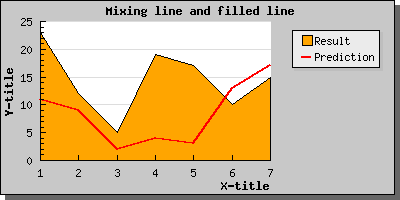Figure 66: Mixing filled and non-filled line plots in the same graph [src]

Let's now go to something a little bit more complicated. How to mix bar and line graphs. Let's just take one of our previous bar graphs and add a line plot to it and see what happens.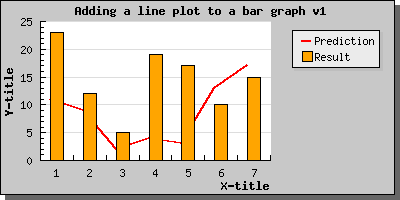Figure 67: Adding a line to a bar graph [src]

Not too bad. But we can't see the line so we change the order in which we add the lines to the graph and sees what happens.Figure 68: Adding a line to a bar graph, in different orderv2 [src]

If you want the line points to be aligned in the center of the bars you can accomplish this is two ways.

If you use a text scale then you need to call the LinePlot::SetBarCenter()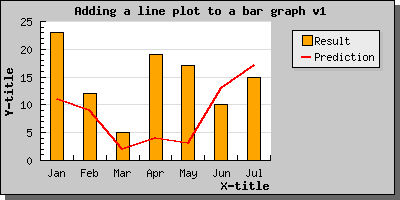Figure 69: Centering the line point in the middle of the bars using LinePlot::SetBarCenter() [src]

You can also use an integer scale. This places both the bar and the line points centered at the tick marks. As the following example will show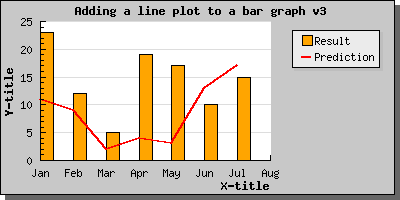Figure 70: Using a linear scale [src]

Note: In this example we also have illustrated the fact that it is possible to add text labels to a linear (or integer) scales as well.

As a final example we show how you can combine a scatter plot and a line plot which could be used to illustrate a regression line fitting.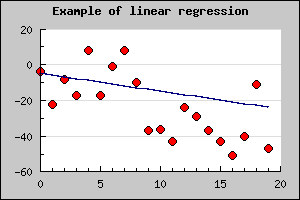Figure 71: Combining a scatter plot and a line plot [src]

Contents Previous Next